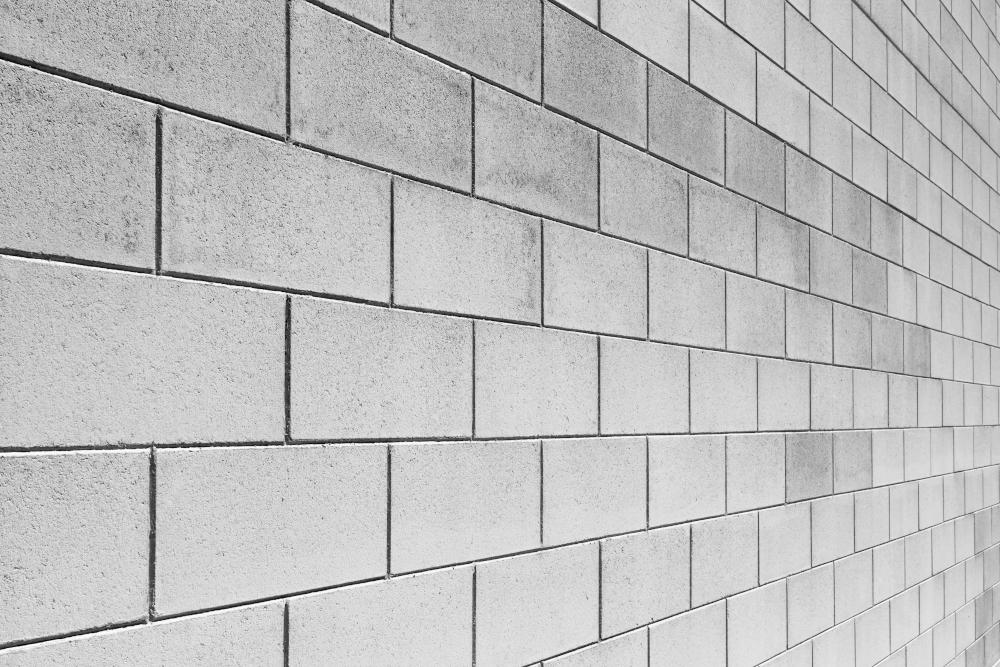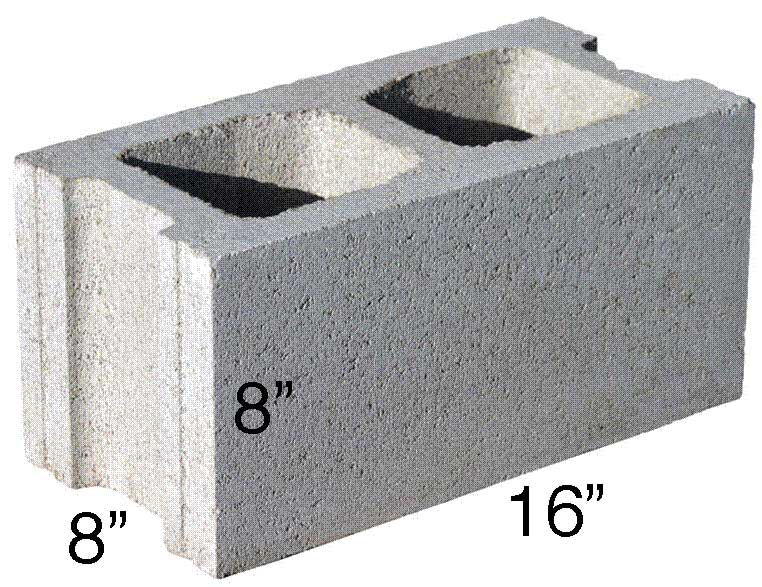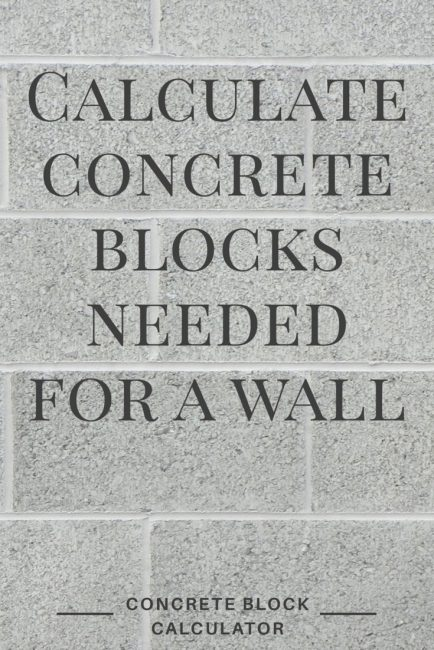# Concrete Block Calculator

Calculate how many concrete blocks and bags of mortar are needed for your project by entering your wall dimensions and block size. Add the block price to get an estimated material price estimate.

Optionally enter the price per block
\$

## Material Estimate:

Blocks

Bags of Mortar

yds3 Sand

### Estimated Material Cost:

Block Cost

Mortar Cost

Sand Cost

Total Cost
Learn how we calculated this below

## How Many Concrete Blocks Do I Need?

Concrete blocks are an affordable building material that can be used for many construction projects and are suited very well for construction walls for foundations or utility buildings.

Concrete blocks typically have holes in the center to reduce the weight, the material needed, and the cost. The average concrete block is composed of cement and coal ash, which is why they are often called cinder blocks.Concrete blocks are concrete masonry units, or CMUs, but we often refer to them as concrete blocks or cinder blocks.

You might be here because you’re wondering how many concrete blocks you’ll need for your project.

A concrete block wall typically requires 1 1/8 blocks per square foot.

Keep reading to learn the process to calculate the number of concrete blocks you need, or just use the calculator above. Simply enter the dimensions of your wall project, and the concrete block calculator will figure out how many blocks you need.

Note: Retaining walls are a bit different from a typical concrete block wall. If you’re building a retaining wall, then try our retaining wall calculator.

## How to Calculate Concrete Blocks

There are several formulas to figure out how many concrete blocks are necessary for a wall project, but you’ll need to start by measuring the wall’s width and height in feet.

### Step One: Find Wall Square Footage

Once you have the wall measurements, calculate the square footage by multiplying the width times the height.

wall sq ft = width × height

### Step Two: Find Block Square Footage

Now you need to figure out the square footage of the block you are using. The standard block size is 16″ wide x 8″ high. Multiply 16×8, and then divide by 144 to get the answer.

block sq ft = 16 × 8 / 144 = 0.89 sq. ft.Different size blocks will have different square footages; apply the formula above to find the solution. Check out our square footage calculator to determine the square footage of your block.

### Step Three: Calculate Blocks

After you have found the square footage of both your wall and your block, determining the number of blocks you need is as simple as dividing the wall square footage by the block square footage.

blocks = wall sq ft / block sq ft

If you’re using a standard 16″ x 8″ x 8″ block, find the number of blocks needed by dividing the wall square footage by 0.89.

blocks = wall sq ft / 0.89

We recommend adding 5%-10% additional blocks to account for broken blocks or blocks that need to be cut for the edges.

Our calculator above may indicate a different quantity of blocks needed since it uses a more precise formula, accounting for partial blocks and cutoffs automatically.

## How to Estimate Mortar for a Block Wall

Calculating the amount of mortar needed for the joints in a concrete block wall will vary depending on the mortar mix you use. On average, it takes about three bags of cement for every 100 blocks.

Divide the number of blocks being installed by 33.3 to calculate how many bags are needed. Standard mortar requires a 1:3 cement to sand mix, which amounts to 1 yd3 of sand for every seven bags of cement.

You can also purchase pre-mixed bags of mortar, which generally yield around 12 blocks per 80-pound bag. Try our mortar calculator to calculate how many bags are needed.

## How to Estimate Grout or Concrete Fill

Estimating the concrete needed to fill the voids in concrete block involves finding the volume of the void in the block being used and multiplying that by the number of voids in the wall. Measure the length, width, and height of the void in inches, then multiply to get the void’s cubic inches.

Multiply the cubic inches by the number of voids in the wall, typically 2 per block. Finally, divide cubic inches by 46,656 to find cubic yards of concrete needed.

Use our block fill calculator to estimate the fill volume.

## Tools Needed to Install a Concrete Block Wall

If you’re installing a concrete block wall, you will need several tools to correctly do the job. Here is a small list of tools that are necessary to build a wall.

•  Brick Trowel: This is used to lay an even bed of mortar when setting block.
•  Mason Line: Use mason line to ensure the wall is straight and level and the block is set at an even height.
•  Level: Make sure you have a level on hand to verify that each block is straight and level with adjacent blocks.
•  Jointer: A jointer will help get smooth, clean joints for a professional look.
•  Tape Measure: You’re definitely going to need a tape measure for this one.
•  Mixing Tub: Mix mortar in a mixing tub to keep your wheelbarrow clean.

As an Amazon Associate we may earn commissions from qualifying purchases from Amazon. Learn more

## Standard Concrete Block Sizes

You’ll need to know the size of the concrete block you’ll be using to determine how many blocks you need for your project. The most common sizes are 8″ high by 8″ wide and 8″ high by 16″ wide in varying thicknesses.

The block’s thickness is not critical to finding how many blocks you need, but it is essential when estimating how much mortar and other masonry material you need. The common thicknesses are 4″, 6″, 8″, 10″, 12″, and 14″.

Refer to the tables below for standard block sizes, including the nominal dimensions. The nominal size is the block’s actual size, while the size includes a 3/8″ mortar joint and is the size that should be used when estimating material.

The standard mortar joint should be 3/8″ thick for your wall.

### 2″ and 3″ CMU Block Dimensions

Chart showing the dimensions of standard 2″ and 3″ blocks and the number of blocks needed per 100 sq ft of wall
Size D x H x W Nominal D x H x W Blocks per 100 ft2
2″ x 8″ x 16″ 1 58” x 7 58” x 15 58 113
3″ x 8″ x 16″ 2 58” x 7 58” x 15 58 113

### 4″ CMU Block Dimensions

Chart showing the dimensions of standard 4″ blocks and the number of blocks needed per 100 sq ft of wall
Size D x H x W Nominal D x H x W Blocks per 100 ft2
4″ x 8″ x 8″ 3 58” x 7 58” x 7 58 226
4″ x 8″ x 16″ 3 58” x 7 58” x 15 58 113

### 6″ CMU Block Dimensions

Chart showing the dimensions of standard 6″ blocks and the number of blocks needed per 100 sq ft of wall
Size D x H x W Nominal D x H x W Blocks per 100 ft2
6″ x 8″ x 8″ 5 58” x 7 58” x 7 58 226
6″ x 8″ x 16″ 5 58” x 7 58” x 15 58 113

### 8″ CMU Block Dimensions

Chart showing the dimensions of standard 8″ blocks and the number of blocks needed per 100 sq ft of wall
Size D x H x W Nominal D x H x W Blocks per 100 ft2
8″ x 8″ x 8″ 7 58” x 7 58” x 7 58 226
8″ x 8″ x 16″ 7 58” x 7 58” x 15 58 113

### 10″ CMU Block Dimensions

Chart showing the dimensions of standard 10″ blocks and the number of blocks needed per 100 sq ft of wall
Size D x H x W Nominal D x H x W Blocks per 100 ft2
10″ x 8″ x 8″ 9 58” x 7 58” x 7 58 226
10″ x 8″ x 16″ 9 58” x 7 58” x 15 58 113

### 12″ CMU Block Dimensions

Chart showing the dimensions of standard 12″ blocks and the number of blocks needed per 100 sq ft of wall
Size D x H x W Nominal D x H x W Blocks per 100 ft2
12″ x 8″ x 8″ 11 58” x 7 58” x 7 58 226
12″ x 8″ x 16″ 11 58” x 7 58” x 15 58 113

### 14″ CMU Block Dimensions

Chart showing the dimensions of standard 14″ blocks and the number of blocks needed per 100 sq ft of wall
Size D x H x W Nominal D x H x W Blocks per 100 ft2
14″ x 8″ x 8″ 13 58” x 7 58” x 7 58 226
14″ x 8″ x 16″ 13 58” x 7 58” x 15 58 113

## How Much Does a Block Wall Cost?

A wall typically costs \$10 – \$15 to install; learn more about a wall project’s cost factors. We suggest getting several professional estimates to get the best labor cost and find the right company for your project.

Check out our concrete calculator to help with your other concrete projects.## References

1. Wikipedia, Concrete Masonry Unit, https://en.wikipedia.org/wiki/Concrete_masonry_unit